# Function tables with two-step rules Online Quiz

Following quiz provides Multiple Choice Questions (MCQs) related to Function tables with two-step rules. You will have to read all the given answers and click over the correct answer. If you are not sure about the answer then you can check the answer using Show Answer button. You can use Next Quiz button to check new set of questions in the quiz.### Explanation

Step 1:

In y = 3x + 1, for x = 0, y = 1; for x = 1, y = 3(1) + 1 = 4; Similarly, for x = 2, y = 7; for x = 3, y = 10 and for x = 4, y =13.

Step 2:

Making a table of x and y values we get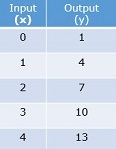### Explanation

Step 1:

In y = 2x − 3, for x = 0, y = −3; for x = 1, y = 2(1) − 3 = − 1; Similarly, for x = 2, y = 1; for x = 3, y = 3 and for x = 4, y = 5.

Step 2:

Making a table of x and y values we get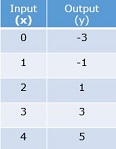### Explanation

Step 1:

In y = 5x − 3, for x = 0, y = −3; for x = 1, y = 5(1) − 3 = 2; Similarly, for x = 2, y = 7; for x = 3, y = 12 and for x = 4, y = 17.

Step 2:

Making a table of x and y values we get### Explanation

Step 1:

In y = 3x − 5, for x = 0, y = −5; for x = 1, y = 3(1) − 5 = −2; Similarly, for x = 2, y = 1; for x = 3, y = 4 and for x = 4, y = 7.

Step 2:

Making a table of x and y values we get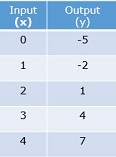### Explanation

Step 1:

In y = 2x + 2, for x = 0, y = 2; for x = 1, y = 2(1) + 2 = 4; Similarly, for x = 2, y = 6; for x = 3, y = 8 and for x = 4, y = 10.

Step 2:

Making a table of x and y values we get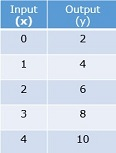### Explanation

Step 1:

In y = 5x + 1, for x = 0, y = 1; for x = 1, y = 5(1) + 1 = 6; Similarly, for x = 2, y = 11; for x = 3, y = 16 and for x = 4, y = 21.

Step 2:

Making a table of x and y values we get### Explanation

Step 1:

In y = 3x − 3, for x = 0, y = −3; for x = 1, y = 3(1) − 3 = 0; Similarly, for x = 2, y = 3; for x = 3, y = 6 and for x = 4, y = 9.

Step 2:

Making a table of x and y values we get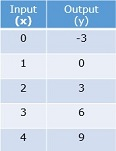### Explanation

Step 1:

In y = 4x + 2, for x = 0, y = 2; for x = 1, y = 4(1) + 2 = 6; Similarly, for x = 2, y = 10; for x = 3, y = 14 and for x = 4, y = 18.

Step 2:

Making a table of x and y values we get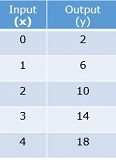### Explanation

Step 1:

In y = 2x − 2, for x = 0, y = − 2; for x = 1, y = 2(1) − 2 = 0; Similarly, for x = 2, y = 2; for x = 3, y = 4 and for x = 4, y = 6.

Step 2:

Making a table of x and y values we get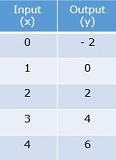### Explanation

Step 1:

In y = 5x − 2, for x = 0, y = − 2; for x = 1, y = 5(1) − 2 = 3; Similarly, for x = 2, y = 8; for x = 3, y = 13 and for x = 4, y = 18.

Step 2:

Making a table of x and y values we get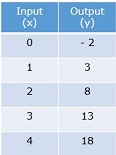function_tables_with_two_step_rules.htm